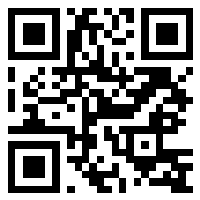## 第一学期青岛版一年级数学1-4单元测试题

2015—2016学年第一学期一年级数学1-4单元测试题

2+5= 3+6= 9-4= 4+4= 3+5=

8-6= 0-4= 6+3= 9-7= 6+3=

2+3+2= 8-4-2= 8+2-6=

8+0-7= 9-5+3= 10-6-0=

1. 填一填，看谁做得最准确。

1.与9相邻的两个数分别是（ ）和（ ）。

2.按顺序填数

（1） 1 ，2 ,（ ），( ), 5 ，（ ）， 7

（2） 9 ， 8 ， （ ）， 6 ，（ ），4

（3） 2， 4，( )，( ），10

3.△ △ △ △ △ △ △ △ △

（1）一共有（ ）个三角形。

（2）把右边的4个圈起来。

（3）把从左边数的第4个三角形涂上颜色。

4.把下列各数按从大到小的顺序排列起来

2 6 10 3 9 0

□ > □ > □> □ > □ > □

5. □ □ □ □ □ □

△ △ △ △

（ ）比（ ）多 □○□

8＋( )＝10 4＋( )＝7 （　　）＋４＝７　　（　　）－９＝０ ( )－4＝5　 2＝( )-6

8○6 5+4○7 7-3 ○ 1+2

10○8 9+0○9 6-2 ○ 6+2

2○6=8 5○4=9

9○2=7 4○4=8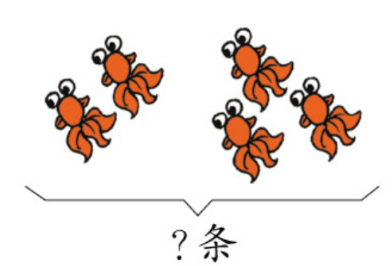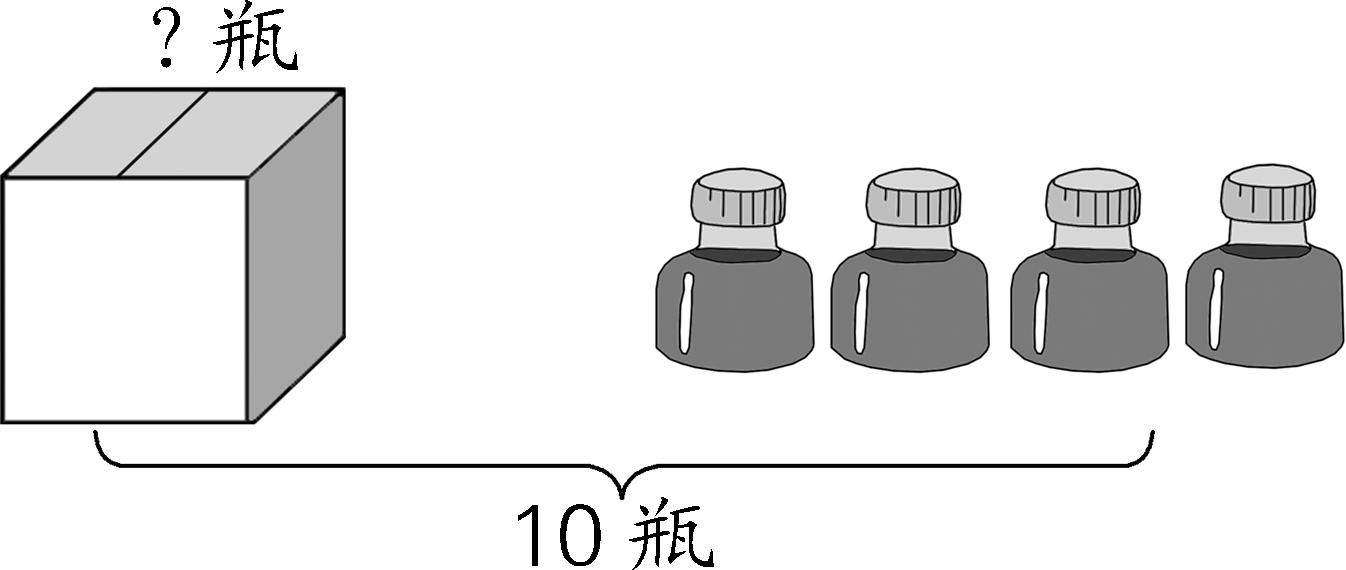1.

□○□=□（瓶） □○□=□（条）

2.

□＋□=□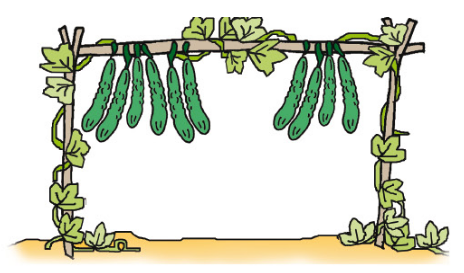□＋□=□

□－□=□

□－□=□

3.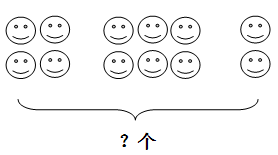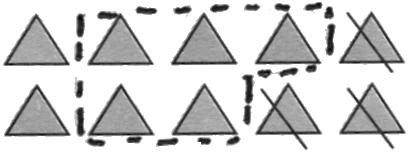＝ ＝

1. 重的下面画“√” 2. 最高的画“√”，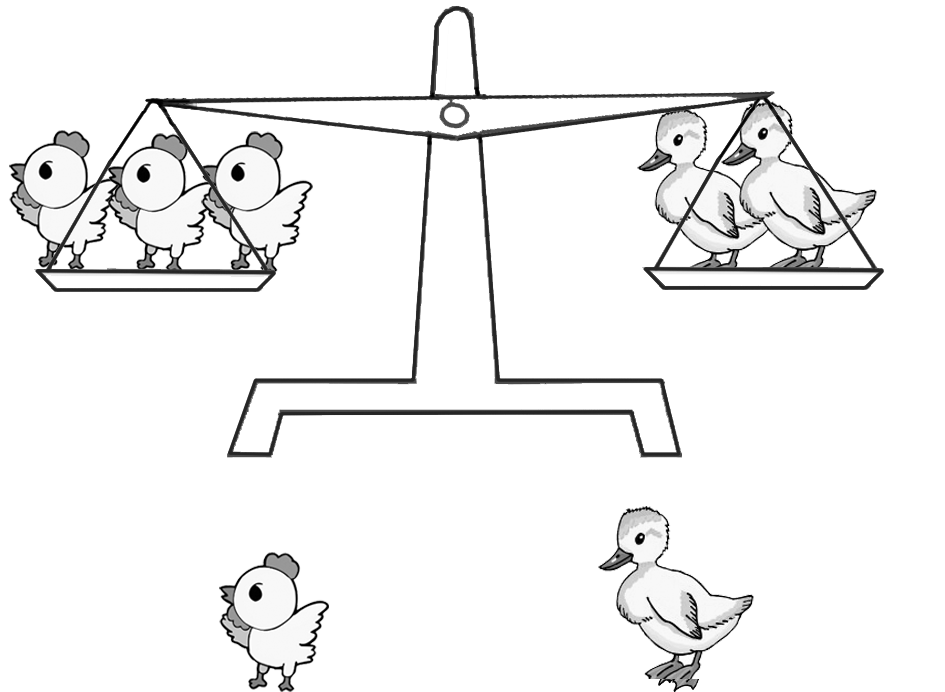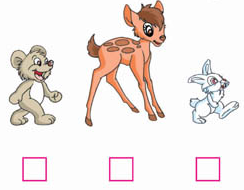□ □

3.长的下面画 “√” 4.高的下面画“√”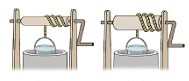□ □ □ □

5.把每行不同类的圈出来。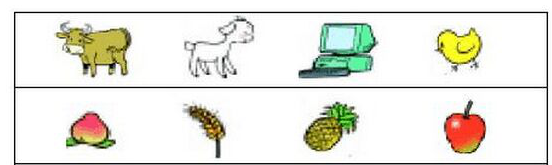1. 2.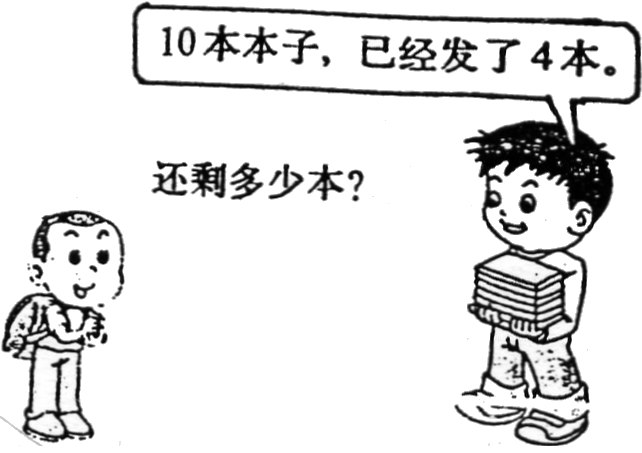=

(人)

=

(本)

3.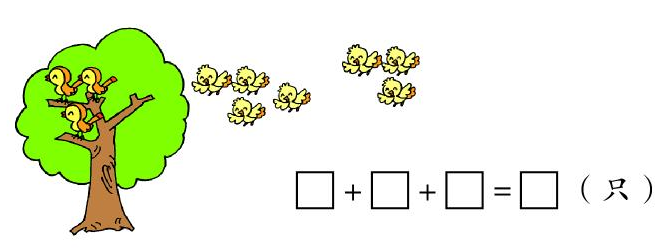4.原来有10个○，划去2个○，又去掉4个○，还剩几个○？

=

(个)